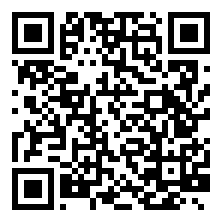HDUOJ 6397 - Character EncodingJimmy Zhang Aug 16, 2018
•$\sum\limits_{i = 1}^{m} x_i = k \ (x_i \in Z, 0 \le x_i < n)$

$1 \le n, m \le 10^5$

$0 \le k \le 10^5$

$\binom{k + m - 1}{m - 1}$\begin{aligned}
|\bigcup\limits_{i = 1}^{3} S_i| &= (|S_1| + |S_2| + |S_3|) - (|S_1 \cup S_2| + |S_1 \cup S_3| + |S_2 \cup S_3|) + |S_1 \cup S_2 \cup S_3| \
& = \sum\limits_{i = 1}^{3}|S_i| - \sum\limits_{1 \le i < j \le 3}|S_i \cup S_j| + \sum\limits_{1 \le i < j < k \le 3} |S_i \cup S_j \cup S_k|
\end{aligned}

$|S_0| = |S_0 \cup S_1 \cup S_2 \cup S_3| - |S_1 \cup S_2 \cup S_3|$

\begin{aligned}
|S_0| & = |\bigcup\limits_{i = 0}^{m} S_i| - |\bigcup\limits_{i = 1}^{m} S_i| \
& = \binom{k + m - 1}{m - 1} - \left[\sum\limits_{i = 1}^{m} |S_i| - \sum\limits_{1 \le i < j \le m} |S_i \cup S_j| + \dots + (-1)^{m + 1}|S_1 \cup \dots \cup S_m| \right]
\end{aligned}

$F§$ 代表从 $m$$x_i$ 中选取 $p$ 个来违反上界限制的种数，那么上述公式可改写为：

\begin{aligned}
|S_0| & = \binom{k + m - 1}{m - 1} - \left[ F(1) - F(2) + \dots + (-1)^{m + 1} F(m) \right] \
& = \binom{k + m - 1}{m - 1} + \sum\limits_{i = 1}^{m} (-1)^i \cdot F(i)
\end{aligned}

\begin{aligned}
x_1 + x_2 + \dots + x_m & = k\
\Rightarrow (x_1 - n) + (x_2 - n) + \dots + (x_p - n) + x_{p + 1} + \dots + x_m & = k - p \cdot n
\end{aligned}

$F§ = \binom{m}{p} \cdot \binom{k - p \cdot n + m - 1}{m - 1}$

This blog is under a CC BY-NC-SA 4.0 Unported License.